# Area involving rectangles and triangles Online Quiz

#### Complete Python Prime Pack

9 Courses     2 eBooks

#### Artificial Intelligence & Machine Learning Prime Pack

6 Courses     1 eBooks

#### Java Prime Pack

9 Courses     2 eBooks

Following quiz provides Multiple Choice Questions (MCQs) related to Area involving rectangles and triangles. You will have to read all the given answers and click over the correct answer. If you are not sure about the answer then you can check the answer using Show Answer button. You can use Next Quiz button to check new set of questions in the quiz.Q 1 - Find the area of the shaded region in the following figure.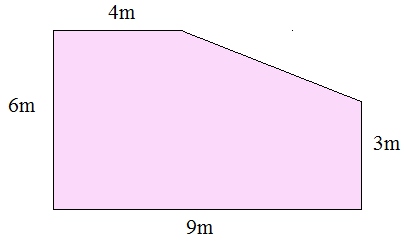### Explanation

Step 1:

Area of rectangle = l × w = 9 × 6 = 54 sq m;

Area of triangle = $\frac{1}{2}$ b h = $\frac{1}{2}$ × 5 × 3 = 7.5 sq m

Step 2:

Area of shaded region = Area of rectangle – Area of triangle

= 54 – 7.5

= 46.5 square m

Q 2 - Find the area of the shaded region in the following figure.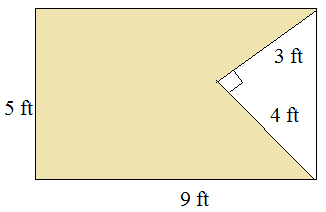### Explanation

Step 1:

Area of rectangle = l × w = 9 × 5 = 45 sq ft;

Area of triangle = $\frac{1}{2}$ b h = $\frac{1}{2}$ × 4 × 3 = 6 sq ft

Step 2:

Area of shaded region = Area of rectangle – Area of triangle

= 45 – 6

= 39 square ft

Q 3 - Find the area of the shaded region in the following figure.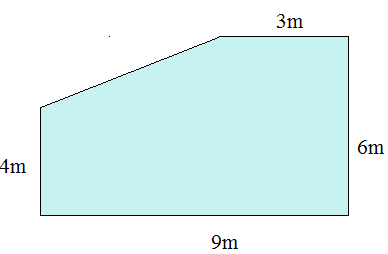### Explanation

Step 1:

Area of rectangle = l × w = 9 × 6 = 54 sq m;

Area of triangle = $\frac{1}{2}$ b h = $\frac{1}{2}$ × 6 × 2 = 6 sq m

Step 2:

Area of shaded region = Area of rectangle – Area of triangle

= 54 – 6

= 48 square m

Q 4 - Find the area of the shaded region in the following figure.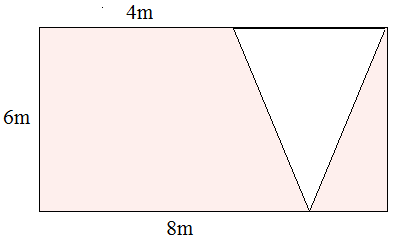### Explanation

Step 1:

Area of rectangle = l × w = 8 × 6 = 48 sq m;

Area of triangle = $\frac{1}{2}$ b h = $\frac{1}{2}$ × 6 × 4 = 12 sq m

Step 2:

Area of shaded region = Area of rectangle – Area of triangle

= 48 – 12

= 36 square m

Q 5 - Find the area of the shaded region in the following figure.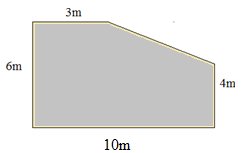### Explanation

Step 1:

Area of rectangle = l × w = 10 × 6 = 60 sq m;

Area of triangle = $\frac{1}{2}$ b h = $\frac{1}{2}$ × 7 × 2 = 7 sq m

Step 2:

Area of shaded region = Area of rectangle – Area of triangle

= 60 – 7

= 53 square m

Q 6 - Find the area of the shaded region in the following figure.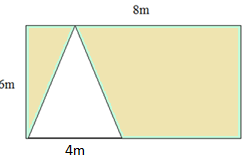### Explanation

Step 1:

Area of rectangle = l × w = 8 × 5 = 40 sq m;

Area of triangle = $\frac{1}{2}$ b h = $\frac{1}{2}$ × 5 × 4 = 10 sq m

Step 2:

Area of shaded region = Area of rectangle – Area of triangle

= 40 – 10

= 30 square m

Q 7 - Find the area of the shaded region in the following figure.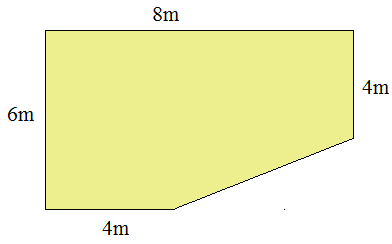### Explanation

Step 1:

Area of rectangle = l × w = 8 × 6 = 48 sq m;

Area of triangle = $\frac{1}{2}$ b h = $\frac{1}{2}$ × 4 × 2 = 4 sq m

Step 2:

Area of shaded region = Area of rectangle – Area of triangle

= 48 – 4

= 44 square m

Q 8 - Find the area of the shaded region in the following figure.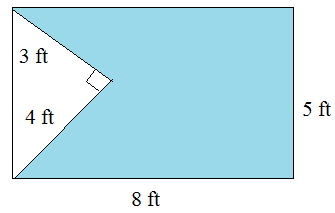### Explanation

Step 1:

Area of rectangle = l × w = 8 × 5 = 40 sq ft;

Area of triangle = $\frac{1}{2}$ b h = $\frac{1}{2}$ × 4 × 3 = 6 sq ft

Step 2:

Area of shaded region = Area of rectangle – Area of triangle

= 40 – 6

= 34 square ft

Q 9 - Find the area of the shaded region in the following figure.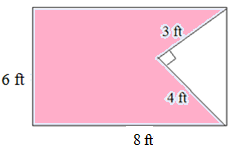### Explanation

Step 1:

Area of rectangle = l × w = 8 × 6 = 48 sq ft;

Area of triangle = $\frac{1}{2}$ b h = $\frac{1}{2}$ × 4 × 3 = 6 sq ft

Step 2:

Area of shaded region = Area of rectangle – Area of triangle

= 48 – 6

= 42 square ft

Q 10 - Find the area of the shaded region in the following figure.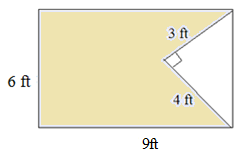### Explanation

Step 1:

Area of rectangle = l × w = 9 × 6 = 54 sq ft;

Area of triangle = $\frac{1}{2}$ b h = $\frac{1}{2}$ × 3 × 3 = 6 sq ft

Step 2:

Area of shaded region = Area of rectangle – Area of triangle

= 54 – 6

= 48 square ft

area_involving_rectangles_and_triangles.htm